# Electronics and Communication Engineering - Automatic Control Systems

Exercise : Automatic Control Systems - Section 5
1.
A phase lead network with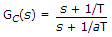where a < 1, gives maximum phase lead at a frequency equal to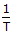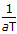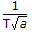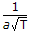Explanation:
No answer description is available. Let's discuss.

2.
Generally a servomotor operates at
high torque and high speed
low torque and low speed
low torque and high speed
high torque and low speed
Explanation:
No answer description is available. Let's discuss.

3.
If C is thermal capacitance, m is mass of liquid and c is specific heat, then
C = mc
c = mC
m = cC
C = (mc)2
Explanation:
No answer description is available. Let's discuss.

4.

Assertion (A): The concept of impulse function is useful in differentiating discontinuous functions.

Reason (R):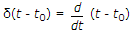Both A and R are correct and R is correct explanation of A
Both A and R are correct but R is not correct explanation of A
A is correct but R is wrong
R is correct but A is wrong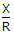and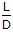are both smallis large butis smallis small butis largeandare both large